# ACI Mix Design Example

A concrete mix is to be designed for use in a 250 mm (10 inch) thick JPCP pavement.  The desired properties are:

• Slump = 25 mm (1.0 inch)
• Average 28-day flexural strength of at least 4.5 MPa (650 psi)
• Coarse aggregate: nominal maximum size = 37.5 mm (1.5 inch), dry-rodded weight = 1600 kg/m3 (100 lb/ft3), specific gravity = 2.68, moisture content = 1.0 percent, absorption = 0.5 percent
• Fine aggregate: fineness modulus = 2.80, specific gravity = 2.64, moisture content = 5 percent, absorption = 0.7 percent
• Air content: 4.5 – 6.5 percent
• Maximum allowable water-cement ratio = 0.44
• Minimum cement content = 335 kg (565 lb)
• Density of water = 1000 kg/m3 (62.4 lb/ft3)
• Specific gravity of portland cement = 3.15 (typically assumed value)

## Slump

Specified as 25 mm (1.0 inch).  This is typical for pavement PCC.

## Maximum Aggregate Size

Specified as 37.5 mm (1.5 inches).  This is well within the general ACI recommendation that the nominal maximum size be limited to 1/3 of slab depth.  No reinforcing steel will be used so the thumbrule pertaining to clear space between reinforcing bars is not applicable.

## Mixing Water and Air Content Estimation

In order to achieve an air content above 2 – 3 percent, PCC must be air-entrained.  Therefore, using the mixing water and air content estimation table, air-entrained PCC with a target 25 mm (1 inch) slump and a 37.5 mm (1.5 inch) nominal maximum aggregate size will require about 148 kg/m3 (250 lb/yd3) of mixing water.  Enough air-entraining admixture will be added to achieve a 5.5 percent air content (in the middle of the specified 4.5 – 6.5 percent band).  Keep in mind that water-reducing admixtures can reduce water requirements by about 5 – 10 percent and several will also increase the entrained air content by about 0.5 – 1 percent.

Converting weight to volume, the recommended volume of mixing water in one cubic meter (one cubic yard) of PCC is 0.148 m3 (4.00 ft3).  The amount of entrained air will be 5.5 percent of the total volume or 0.055 m3 (1.49 ft3).

## Water-Cement Ratio

Since the specified strength is flexural strength, a conversion factor must be used to obtain an approximate compressive strength in order to use the water-cement ratio vs. compressive strength table.  A good rule-of-thumb (as used in the ACI Code) is: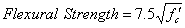where: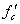equals compressive strength

Solving for compressive strength, we get about 51.7 MPa (7,500 psi).  This value is not listed in Table 17 for determining water-cement ratios.  Therefore, a more involved determination of the water-cement ratio is needed.  Water-cement ratios may also be determined using local experience.  Keep in mind that Table 4 is rarely used in pavement PCC mix design.  Additionally, ACI recommends a maximum water-cement ratio for PCC exposed to freezing and thawing in moist conditions (such as curbs and gutters) or PCC in the presence of deicing chemicals (such as PCC pavement slabs) of 0.45.  In this instance, assume that local experience dictates a water-cement ratio of around 0.39.

## Cement Content

Based on the previous mixing water content and water-cement ratio, cement content is calculated to be: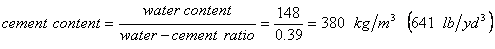The recommended volume of portland cement in one cubic meter (one cubic yard) of PCC is:

(Metric) (English)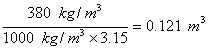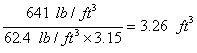## Coarse Aggregate Content

Using the coarse aggregate content table with the given nominal maximum aggregate size of 37.5 mm (1.5 inches) and the given fine aggregate fineness modulus of 2.80, the recommended volume fraction of coarse aggregate is 0.71.  This means that the coarse aggregate should occupy 71 percent of the total volume.  However, this volume of aggregate includes the volume of air between the aggregate particles.  Therefore, this 71 percent volume must be converted to a weight of aggregate.  Given the 1600 kg/m3 (100 lb/ft3) dry-rodded unit weight, this equates to:

(Metric) (English)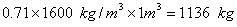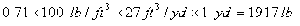Now, the recommended volume of coarse aggregate (which is now only the solid volume of coarse aggregate) in one cubic meter (one cubic yard) of PCC is:
(Metric) (English)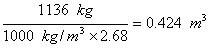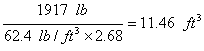## Fine Aggregate Content

The fine aggregate content can be found by subtracting the other constituent volumes from the unit volume:

(Metric) (English)
Unit volume (1 m3 or yd3) 1.000 m3 27.00 ft3
- Volume of mixing water - 0.148 m3 4.00 ft3
- Volume of air - 0.055 m3 1.49 ft3
- Volume of portland cement - 0.121 m3 3.26 ft3
- Volume of coarse aggregate - 0.424 m3 11.46 ft3
equals Volume of fine aggregate equals 0.252 m3 6.79 ft3

Therefore, the weight of fine aggregate per unit volume is:

(Metric) (English)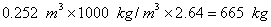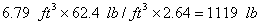## Adjustments for Aggregate Moisture

Since there is moisture in both the coarse and fine aggregate their trial batch weights must be adjusted.

(Metric) (English)
Coarse aggregate: 1136 x 1.01 = 1147 kg/m3 1917 x 1.01 = 1936 lb/yd3
Fine aggregate: 665 x 1.05 = 698 kg/m3 1119 x 1.05 = 1175 lb/yd3

Also, the amount of mixing water needs to be adjusted because both the coarse and fine aggregate are wet and will contribute water to the cement paste.
(Metric) (English)
Coarse aggregate: 1136 x (0.01 - 0.005) = 5.7 kg/m3 1917 x (0.01 - 0.005) = 10 lb/yd3
Fine aggregate: 665 x (0.05 - 0.007) = 28.6 kg/m3 1119 x (0.05 - 0.007) = 48 lb/yd3

Therefore, the amount of mixing water to be added is:
 148 kg - 5.7 kg - 28.6 kg equals 113.7 kg 250 lb - 10 lb - 48 lb equals 192 lb

## Summary

The final trial batch amounts per unit volume (1 m3 or yd3) are:

(Metric) English)
Mixing water 114 kg 192 lb
Portland cement 380 kg 641 lb
Coarse aggregate 1147 kg 1936 lb
Fine aggregate 698 kg 1175 lb

Usually, to make trial batches, something less than the unit volume is made – a typical trial batch size is  0.03 m3 (1 ft3).  Once the trial batch is made it can be tested for slump, air content, flexural strength, compressive strength and any other required property.